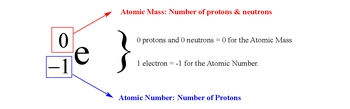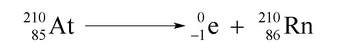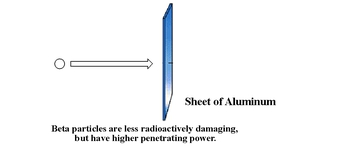Start typing, then use the up and down arrows to select an option from the list.# GOB Chemistry

Learn the toughest concepts covered in your GOB - General, Organic, and Biological Chemistry class with step-by-step video tutorials and practice problems.

Nuclear Chemistry

A beta decay or beta emission occurs when an unstable nucleus ejects a beta particle to create a new element.

## Understanding Beta Decay

A beta particle has no atomic mass and is represented by an electron.1

#### concept

Beta Decay Concept 11m
Play a video:
Hey, guys, In this new video, we're gonna take a look at beta decay. Now, we're gonna say that beta decay occurs when an unstable nucleus somehow emits a new electron. Now we're gonna say beta particle can be represented by E for the electron. I like trying here. We're gonna say much smaller than the other two subatomic particles. So the atomic mass can just be understood as zero. And here we're gonna say it. Atomic number is negative one because the atomic number is basically the number of protons. Since this is an electron, we're going to say that it's the opposite of a proton, which is one so an electron is negative one. Now, we're gonna say here beta decay can be represented when we emit a beta particle. So, for example, if we had Mercury and remember the 21 means that's its atomic math. So let's say we had mercury to one. If we look on our periodic table, Mercury has an atomic number off 80. We're gonna emit a beta particle. Now, remember, you're gonna say you're atomic masses have t equal each other on both sides of the arrow and your atomic numbers as well. Here. The electron has no mass. So the new element is gonna have a mass up to one. And then here we have to be very careful here. This is minus one. So minus one plus what gives me 80. Well, the answer would have to be 81 because 81 minus one gives me the 80 that I had originally. So just remember what? Remember that. So here would be t l. So this would be an example of a beta decay or a beta emission.2

#### concept

Beta Decay Concept 242s
Play a video:
Now we're gonna say in terms of size, we know that the Alfa particles are the largest radioactive particle. So we're gonna say beta particles, therefore are smaller than alfa particles now, because they're smaller in size, they're gonna be less damaging if we ingest them. So here they're gonna have a lower ionizing power. But the problem here is because they're smaller, they're able to better penetrate into our skin. So here they're gonna have mawr penetrating power. And so to stop, you need a sheet of metal or large block of wood toe. Make sure beta particle does not enter inside of our bodies.

Beta particles are smaller in size, and therefore have more penetrating power. Luckily, they are less radioactively damaging because of their lower ionizing power.3

#### example

Beta Decay Example 11m
Play a video:
So here we have to write the balance Nuclear equations for each of the following beta emissions again. Beta decay. Beta emissions means that the beta particle will be a product. If they had said baited it capture or beta absorption, then it would be a reacted. So here we're starting out with magnesium 25. So that's the atomic mass. On our periodic table. Magnesium has an atomic number off 12. It's gonna emit a beta particle. So our new element that's being created would still have the same atomic mass. And then here, negative one. Plus what number gives me 12. It has to be 13 because 13 minus one gives me the 12 I started out with initially. So this element would be aluminum. Now, Ruffini, Um, are you one? 02 We're gonna see if we look in our periodic table roof in. IAM has an atomic number off 44. Again, we're gonna emit a beta particle, so this number stays 102 And this number here would have to be 45. So it goes upto Rh. Okay, so those were the examples off beta decays or beta emissions
4

#### example

Beta Decay Example 26m
Play a video: